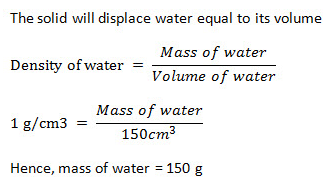Courses

# Test: Archimedes Principle

## 15 Questions MCQ Test Science Class 9 | Test: Archimedes Principle

Description
This mock test of Test: Archimedes Principle for Class 9 helps you for every Class 9 entrance exam. This contains 15 Multiple Choice Questions for Class 9 Test: Archimedes Principle (mcq) to study with solutions a complete question bank. The solved questions answers in this Test: Archimedes Principle quiz give you a good mix of easy questions and tough questions. Class 9 students definitely take this Test: Archimedes Principle exercise for a better result in the exam. You can find other Test: Archimedes Principle extra questions, long questions & short questions for Class 9 on EduRev as well by searching above.
QUESTION: 1

### An object weighing 5 N in air, weighs 4.5 N a liquid. The buoyant force experienced by the object is

Solution:

The buoyant force experienced by the object is:- The buoyant force is in opposite direction to the weight of the object. Hence, if the object weighs 4.5 N in liquid then 0.5 N buoyant force will act on the object.

QUESTION: 2

### The same body is immersed in two liquids A and B in succession. The extent to which the body sinks in liquid B is less then in liquid A. What are the conclusions that could be derived from such an observation

Solution:

The density of liquid B is more than the liquid A as more upthrust is exerted on the body in liquid B and hence it sinks less.

QUESTION: 3

### A sample of milk diluted with water has a density of 1040 kgm-3 . If pure milk has a density of 1080 kgm-3. The percentage of water by volume in milk is

Solution:

Density of pure milk = 1080 Kg /m³
1080 kg / m³ = 100% pure milk
Density of diluted milk = 1032 kg / m³
1032 Kg/m³ = ? % of milk
1032 x 100/1080 = 95.56% of milk
Thus, the percentage of water by volume in milk is 100 – 95.56 = 4.44%

QUESTION: 4

A student lowers a body in a liquid filled in a container. He finds that there is a maximum apparent loss in weight of the body when

Solution:
QUESTION: 5

The density of copper is 9 g/cm3. Then the volume of 90 g copper is​

Solution:

Density of copper = 9 gm/cm^3
Mass of copper  =  90 g
We know;  Density =  mass / volume
⇒ volume  = mass / density
⇒    90 / 9
⇒ Volume  = 10 cm3

QUESTION: 6

The sea water is denser than fresh water due to

Solution:
QUESTION: 7

Instrument used for measuring the density or relative density of liquids is known as

Solution:
QUESTION: 8

A packet of 400 g and volume 200 cm3 is put in a water tank. The relative density of packet is

Solution:
QUESTION: 9

An object of weight 500 N is floating in a liquid. The magnitude of buoyant force acting on it is

Solution:
As we know that the buoyant force acting on a floating body in a liquid is equal to the weight of the floating body,
Buoyant force acting on the body of weight 200N floating in  liquid = 200N
QUESTION: 10

If relative density is 11.3, the density of the substance in SI units is

Solution:
QUESTION: 11

If the density of the object placed in a liquid is equal to the density of the liquid, the object will:

Solution:

If the density of the object placed in liquid is equal to the density of the liquid, then :- It would float submerged in the liquid. Some part of the solid would be above the liquid surface and part would be inside the liquid surface.

QUESTION: 12

The mass of water displaced by a solid of mass 400 g and volume 150 cm3 is​

Solution:QUESTION: 13

Three objects A, B, and C having density 1.12 g cm-3, 0.92 g cm-3 and 2.02 g cm-3 respectively, are placed in a water tank. Which object will sink in water tank?​

Solution:
QUESTION: 14

When a solid body is fully immersed in a liquid, the volume of the displaced liquid is:

Solution: According to Archimedes’ principle, the volume of liquid displaced is equal to the volume of the solid.
QUESTION: 15

If we want to determine the volume of a solid by immersing it in water, the solid should be

Solution:
It should be heavier than water and insoluble in it, so that it sinks and displaces the water and also it does not mix in the water.